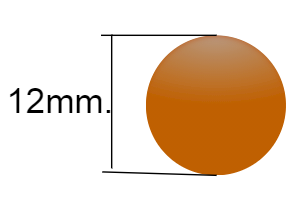### How to calculate the weight of reinforcement bars?/What is D2/162 in bar weight calculation formula?

For the calculation procedure, go through the following steps.👇

The weight of the reinforcement bar is calculated by the formula

= [volume × density.]Reinforcement bar.

Now, for the calculation purpose, let us consider the rebar of 12mm. diameter, having 1m. length.12mm. dia. rebar.

Here, length L = 1m. diameter D = 12mm. and the density of steel is 7850kg/m3Rebar of 1m. length

The volume of the rebar

= [cross-sectional area × length]

= [π ×( D2)/4 × L]

= [3.142 × ( 0.012 × 0.012) /4 × 1]

= [3.142 × .000036 × 1]

= 0.000113 cum.

Weight of the rebar

= [volume × density]

= [0.000113 × 7850]

### Alternate method:

Weight of the rebar is given by the formula

= [(D2) /162]

= [( 12 × 12 ) /162]

=144 / 162

= 0.888kg /meter.

In the second method, we have calculated the weight easily, but the question that comes to our mind is,

### What is 162 in the reinforcement bar weight calculation formula?

Now, we will do the following step to derive that formula.

As you know,

Weight of the rebar

= [cross-sectional area × length × density]

= [π ×( D2/4) × L × 7850]

= [3.142 × ( D2/4) × (1×1000 )× 7850/(1000×1000×1000)]

Here, we have converted length and density into the mm. unit, as the diameter of the rebar is taken in the mm.

= [3.142 D2/4 × (0.00785)]

= [0.006166 D2]

Inversing 0.006166 in the above equation, we get

Weight of the rebar

=  D2/162.18 per meter length.

Note:   The diameter of the rebar should be taken in mm. while calculating the weight.

,

#### 1 comment:

1.Very best 👍